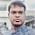# Digital "Square root" Computation of a number

By Popular Demand!

input clk,start;
input [7:0] data;

output done;

reg busy;

reg [1:0] bit;

wire [3:0] trial;

assign trial = answer | (1 << bit);

always @ (posedge clk)
begin
if (busy)
begin
if (bit == 0) busy <= 0;
else
bit <= bit - 1;

if (trial*trial <= data)
end
else if (start)
begin
busy <= 1;

bit <= 3;
end
end

assign done
= ~busy;
endmodule

1.So your solution is trial and error? This seems terribly inefficient, especially for hardware.

2.3.(Step1) Input N

(Step2) Set XL=1, and XH=N

(Step3) Set XM = (XL+XH) / 2 (Round if necessary)

(Step4) If XH-XL < 2 Then

STOP. Result is XM

(Step5) If XM * XM > N Then

set XH = XM

else

set XL = XM

(Step 6) Goto Step3

i have this algorithm, how i can impelent it in verilog? please can you help?